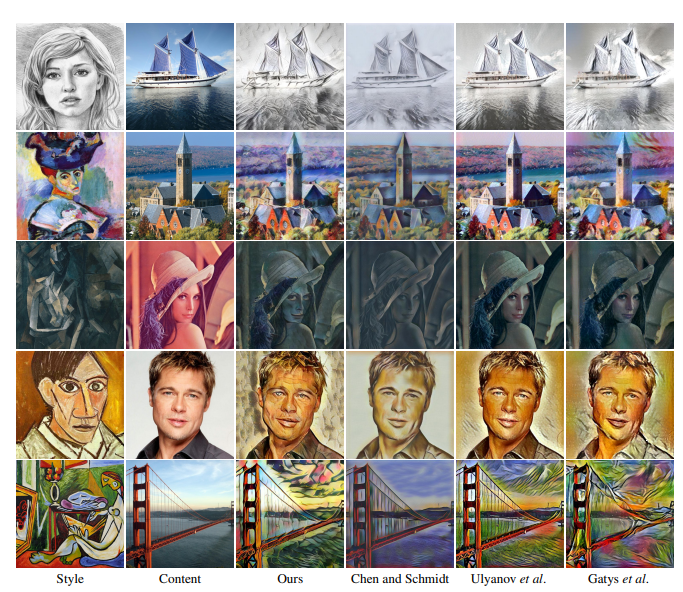# Source

Paper: [ICCV’2017] https://arxiv.org/abs/1703.06868

Authors: Xun Huang, Serge Belongie

# Contributions

In this paper, the authors present a simple yet effective approach that for the first time enables arbitrary style transfer in real-time.

Arbitrary style transfer: takes a content image $C$ and an arbitrary style image $S$ as inputs, and synthesizes an output image with the same content as $C$ and the same syle as $S$.# Background

## Batch Normalization

Given a input batch $x \in \mathbb{R}^{N \times C \times H \times W}$, batch normalization (BN) normalizes the mean and standard deviation for each individual feature channel:

$$\mathrm{BN}(x)=\gamma\left(\frac{x-\mu(x)}{\sigma(x)}\right)+\beta$$

where $\gamma , \beta \in \mathbb{R}^{C}$ are affine parameters learned from data. $\mu(x) , \sigma(x) \in \mathbb{R}^{C}$ are mean and standard deviation computed across batch size and spatial dimensions, independently.

$$\mu_{c}(x)=\frac{1}{N H W} \sum_{n=1}^{N} \sum_{h=1}^{H} \sum_{w=1}^{W} x_{n c h w}$$

$$\sigma_{c}(x)=\sqrt{\frac{1}{N H W} \sum_{n=1}^{N} \sum_{h=1}^{H} \sum_{w=1}^{W}\left(x_{n c h w}-\mu_{c}(x)\right)^{2}+\epsilon}$$

## Instance Normalization

Original feed-forward stylization method  utilizes BN layers after the convolutional layer. Ulyanov et al.  found using Instance Normalization (IN) can achiever better stylization.

 D. Ulyanov, V. Lebedev, A. Vedaldi, and V. Lempitsky. Texture networks: Feed-forward synthesis of textures and stylized images. In ICML, 2016.  D. Ulyanov, A. Vedaldi, and V. Lempitsky. Improved texture networks: Maximizing quality and diversity in feed-forward stylization and texture synthesis. In CVPR, 2017.

From , the authors said: "A simple observation that may make learning simpler is that the result of stylization should not, in general, depend on the contrast of the content image but rather should match the contrast of the texture that is being applied to it. Thus, the generator network should discard contrast information in the content image. We argue that learning to discard contrast information by using standard CNN building block is unnecessarily difficult, and is best done by adding a suitable layer to the architecture."

The Instance Normalization (IN) layer is defined as:

$$\operatorname{IN}(x)=\gamma\left(\frac{x-\mu(x)}{\sigma(x)}\right)+\beta$$

Different from BN layers, here $\mu(x)$ and $\sigma(x)$ are computed across spatial dimensions independently for each channel and each sample:

$$\mu_{n c}(x)=\frac{1}{H W} \sum_{h=1}^{H} \sum_{w=1}^{W} x_{n c h w}$$

$$\sigma_{n c}(x)=\sqrt{\frac{1}{H W} \sum_{h=1}^{H} \sum_{w=1}^{W}\left(x_{n c h w}-\mu_{n c}(x)\right)^{2}+\epsilon}$$ Another difference is that IN layers are applied at test time unchanged, whereas BN layers usually replace minibatch statistics with population statistics.

## Conditional Instance Norm

Dumoulin et al.  proposed a conditional instance normalization (CIN) layer that learns a different set of parameters $\gamma^{s}$ and $\beta^{s}$ for each style $s$

$$\operatorname{CIN}(x ; s)=\gamma^{s}\left(\frac{x-\mu(x)}{\sigma(x)}\right)+\beta^{s}$$

 V. Dumoulin, J. Shlens, and M. Kudlur. A learned representation for artistic style. In ICLR, 2017.

The paper argues that instance normalization performs a form of style normalization by normalizing feature statistics, namely the mean and variance.

The reason behind the success of conditional instance normalization also becomes clear: different affine parameters can normalize the feature statistics to different values, thereby normalizing the output image to different styles.

AdaIN receives a content input $x$ and a style input $y$, and simply aligns the channelwise mean and variance of $x$ to match those of $y$.

$$\operatorname{AdaIN}(x, y)=\sigma(y)\left(\frac{x-\mu(x)}{\sigma(x)}\right)+\mu(y)$$ in which we simply scale the normalized content input with $\sigma(y)$, and shift it with $\mu(y)$. Similar to IN, these statistics are computed across spatial locations.

• Explanation: Intuitively, let us consider a feature channel that detects brushstrokes of a certain style. A style image with this kind of strokes will produce a high average activation for this feature. The output produced by AdaIN will have the same high average activation for this feature, while preserving the spatial structure of the content image. The brushstroke feature can be inverted to the image space with a feed-forward decoder. The variance of this feature channel can encoder more subtle style information, which is also transferred to the AdaIN output and the final output image.

In short, AdaIN performs style transfer in the feature space by transferring feature statistics, specifically the channel-wise mean and variance.

# Training

## Network Structure

We adopt a simple encoder-decoder architecture, in which the encoder $f$ is fixed to the first few layers (up to relu4 1) of a pre-trained VGG-19. After encoding the content and style images in feature space, we feed both feature maps to an AdaIN layer that aligns the mean and variance of the content feature maps to those of the style feature maps, producing the target feature maps $t$:$$t=\operatorname{AdaIN}(f(c), f(s))$$

A randomly initialized decoder g is trained to map $t$ back to the image space, generating the stylized image $T(c, s)$.

$$T(c, s)=g(t)$$

## Loss Function

It uses the common loss with the pre-trained VGG-19 to compute the loss function to train the decoder.

$$\mathcal{L}=\mathcal{L}_c+\lambda \mathcal{L}_s$$

which is a weighted combination of the content loss $\mathcal{L}_c$and the style loss $\mathcal{L}_s$ with the style loss weight $\lambda$. The content loss is the Euclidean distance between the target features and the features of the output image.

• Content loss $\mathcal{L}_c$: We use the AdaIN output $t$ as the content target, instead of the commonly used feature responses of the content image. We find this leads to slightly faster convergence and also aligns with our goal of inverting the AdaIN output $t$.

$$\mathcal{L}_{c}=|f(g(t))-t|_2$$

• Style loss $\mathcal{L}_s$: Although we find the commonly used Gram matrix loss can produce similar results, we match the IN statistics because it is conceptually cleaner.
$$\mathcal L_ s=\sum_{i=1}^{L}\left\|\mu\left(\phi_{i}(g(t))\right)-\mu\left(\phi_{i}(s)\right)\right\|_{2}+ \sum_{i=1}^{L}\left\|\sigma\left(\phi_{i}(g(t))\right)-\sigma\left(\phi_{i}(s)\right)\right\|_{2}$$

where each $\phi_i$ denotes a layer in VGG-19 used to compute the style loss. In our experiments we use relu1 1, relu2 1, relu3 1, relu4 1 layers with equal weights.

# Experiments

• Quanlitative Comparison• Quantitative evaluations Average content and style loss• speed test

• ablation study

## Send CommentEdit Comment

|´・ω・)ノ
ヾ(≧∇≦*)ゝ
(☆ω☆)
（╯‵□′）╯︵┴─┴
￣﹃￣
(/ω＼)
∠( ᐛ 」∠)＿
(๑•̀ㅁ•́ฅ)
→_→
୧(๑•̀⌄•́๑)૭
٩(ˊᗜˋ*)و
(ノ°ο°)ノ
(´இ皿இ｀)
⌇●﹏●⌇
(ฅ´ω`ฅ)
(╯°A°)╯︵○○○
φ(￣∇￣o)
ヾ(´･ ･｀｡)ノ"
( ง ᵒ̌皿ᵒ̌)ง⁼³₌₃
(ó﹏ò｡)
Σ(っ °Д °;)っ
( ,,´･ω･)ﾉ"(´っω･｀｡)
╮(╯▽╰)╭
o(*////▽////*)q
＞﹏＜
( ๑´•ω•) "(ㆆᴗㆆ)

Emoji